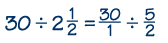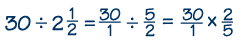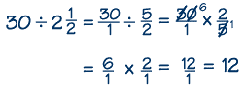Home    |    Teacher    |    Parents    |    Glossary    |    About UsDividing mixed numbers is very similar to multiplying mixed numbers. You just add one step—after changing the divisor into an improper fraction, you then find its reciprocal and multiply. Let's work through a "word problem" example.

The SuperQuik Market has just installed new scanners for its check-out lanes. They claim the average time to check out a customer is 2 ½ minutes. How many customers, on average, can they check out in half an hour?

To solve this problem, we have to know that half an hour is the same as 30 minutes. Then we can divide 30 by 2 ½.

First step: Write the whole number and the mixed number as improper fractions.Second step: Write the reciprocal of the divisor, 2/5, and multiply.Third step: Simplify, if possible. Notice that we can simplify our problem at this step, to make our calculations easier. Five goes evenly into 30, so we can divide both 5 and 30 by 5, to give 1 and 6.Fourth step: Perform the simple multiplication of the numerators and the denominators. We find that the market can check out 12 customers in 30 minutes with its new scanners.

Fifth step: Put the answer in lowest terms, and check the answer. Our answer is already in lowest terms, so there is nothing left to do but check the answer, to be sure it makes sense. We can use estimation and rounding to do our check. If we round 2 ½ minutes to 3 minutes and divide 3 into 30, we get 10 customers in 30 minutes. So it is reasonable that 2 more customers per half hour, or 12 customers, can be checked, since 2 ½ minutes per customer is less than 3 minutes per customer.

 Homework Help | Pre-Algebra | FractionsEmail this page to a friendSearch·  Definitions·  Reducing fractions·  Adding and subtract-     ing fractions·  Multiplying fractions·  Dividing fractions·  Adding and subtract-     ing mixed numbers·  Multiplying     mixed numbers·  Dividing     mixed numbersFirst Glance In Depth Examples WorkoutDividing mixed numbers